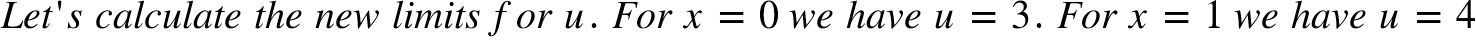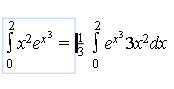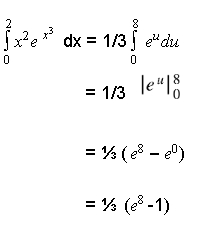Friday, May 31, 2019

The definite integral and the integration by substitution

Objective:

Apply the method of integration by substitution to the resolution of definite integrals

Method (Recall)

The method by substitution consists in substituting the given variable by another variable
that makes the integral easier to calculate.

Let’s integrateby substitution.

If this integral can be writtenwithandthenThe integralbecomes easier to calculate. In the case of a definite integral
we have to calculate the new limits of integration for u.

Example I

Let’s calculate======Example II EvaluateWe can rewrite the expression as:

We have here 2 separate expressionsand x²

We seek to find out if the expression closed to dx is the derivative of the expression x³ in.

We notice that is almost the derivative of . It misses the term 3. Therefore we have
to multiply and divide by 3 in order to have 3 . The given expression becomes:represents a function composite of the exponential function f(x) =and the function g(x) =

We can write=  f(g(x)). 3x represents the derivative of x³. We can write   3x².= . g'(x)

Then we have:

If we do a change of variable by writing u = x³ du/dx = 3x² du = 3x²dx.= g'(x)dx

f(g(x)) =. becomes f(u) = .. There are new limits of integration for the new function of u

In the expresion u = x³, for x = 0 u = 0, for x = 2 u = (2)³ = 8

Let's substitute f(g(x)) by f(u) and g'(x)dx by du:

Let's substitute f(u) by:N.B. We have solved the second example by writing the given function as a composite function. We can skip these steps by doing the change of variable right away.as it was done in the first example,

Practice